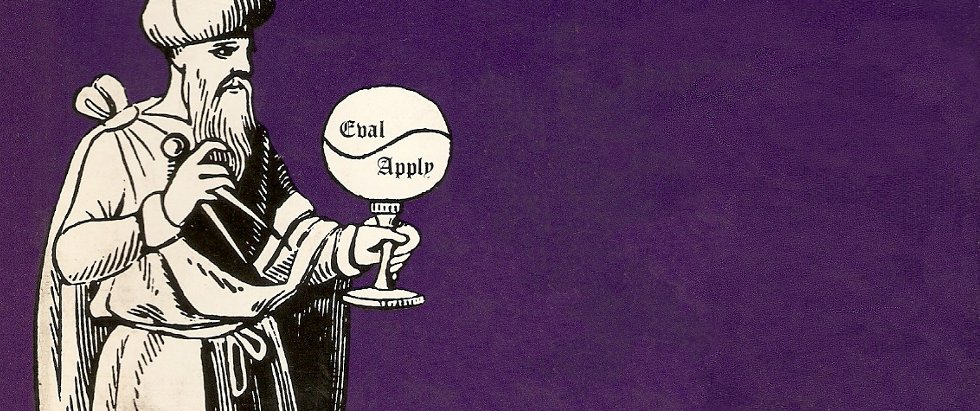# SICP Goodness - Stream (5)

Infinite StreamsDo you think Computer Science equals building websites and mobile apps?

Are you feeling that you are doing repetitive and not so intelligent work?

Are you feeling a bit sick about reading manuals and copy-pasting code and keep poking around until it works all day long?

Do you want to understand the soul of Computer Science?

Streams can also be infinite, let’s look at some examples.

## Integers Stream

We can define all the integers as an infinite stream starting from 1.

(define (integers-starting-from n)
(cons-stream n (integers-starting-from (+ n 1))))

(define integers (integers-starting-from 1))

Let’s try to test it:

1 ]=> (stream-ref integers 2)

;Value: 3

1 ]=> (stream-ref integers 1)

;Value: 2

1 ]=> (stream-ref integers 100)

;Value: 101

Now we have integers, we can then go further and define all the integers that are not divisible by 7:

(define (divisible? x y) (= (remainder x y) 0))

(define no-sevens
(stream-filter
(lambda (x) (not (divisible? x 7)))
integers))

Let’s try it out:

1 ]=> (stream-ref no-sevens 0)

;Value: 1

1 ]=> (stream-ref no-sevens 1)

;Value: 2

1 ]=> (stream-ref no-sevens 6)

;Value: 8

## Infinite Fibonacci Stream

Interestingly, Fibonacci numbers can also be defined as an infinite stream:

(define (fib-gen a b)
(cons-stream a (fib-gen b (+ a b))))

(define fibs (fib-gen 0 1))

Let’s test it out:

1 ]=> (stream-ref fibs 2)

;Value: 1

1 ]=> (stream-ref fibs 3)

;Value: 2

1 ]=> (stream-ref fibs 4)

;Value: 3

1 ]=> (stream-ref fibs 5)

;Value: 5

## Defining Streams Implicitly

Previously, infinite streams are defined using procedures. For example, integers is defined by executing (integers-starting-from 1). Another way to do it is to define stream in terms of itself.

For example, we can define an infinite stream of 1s.

(define ones (cons-stream 1 ones))

Let’s make sure that this works:

1 ]=> (stream-ref ones 0)

;Value: 1

1 ]=> (stream-ref ones 1)

;Value: 1

1 ]=> (stream-ref ones 100)

;Value: 1

Let’s implement a procedure to add two streams.

(define (add-streams s1 s2)
(stream-map + s1 s2))

Notice that the stream-map here is the generic version from Exercise 3.50.

Let’s test this by creating a stream of 2s.

1 ]=> (define twos (add-streams ones ones))

;Value: twos

1 ]=> (stream-ref twos 1)

;Value: 2

1 ]=> (stream-ref twos 100)

;Value: 2

## Define integers and fibs implicitly

We can define integers stream implicitly:

(define integers (cons-stream 1
(add-streams ones integers)))

Also define fibs implicitly:

(define fibs (cons-stream 0
(cons-stream 1
fibs))))